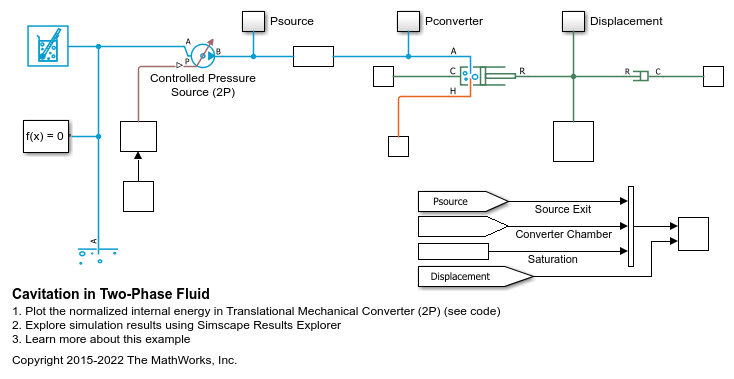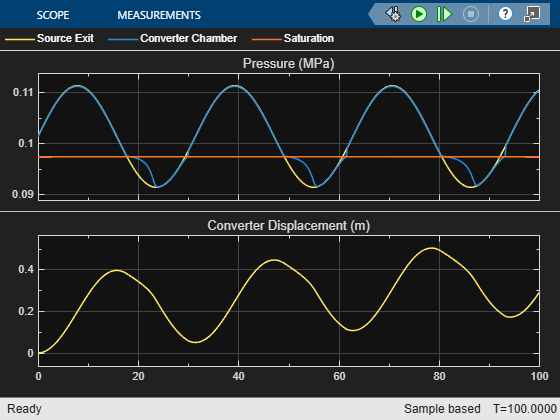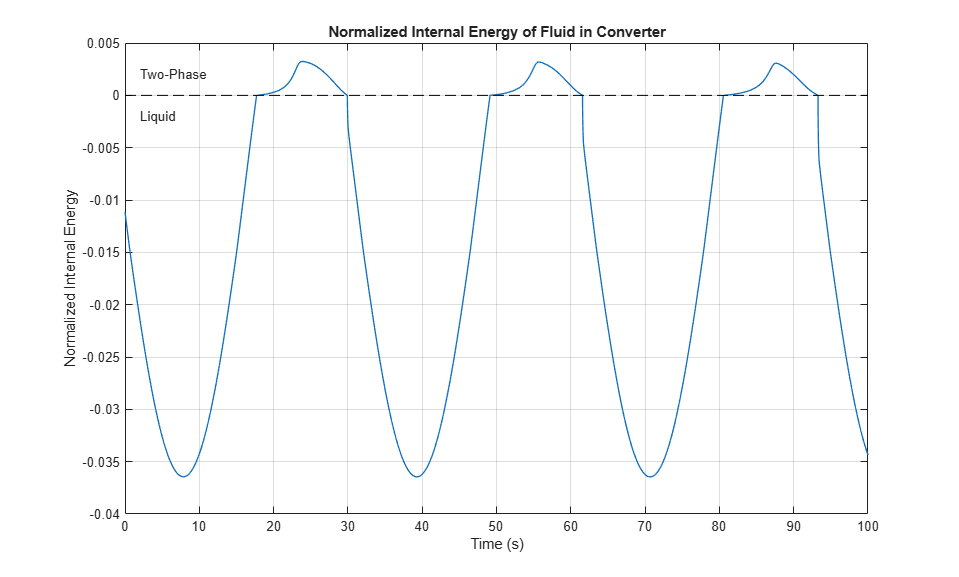# Cavitation in Two-Phase Fluid

This example shows how two-phase fluid components can be used to simulate cavitation. The model is a translational mechanical converter driven by an oscillating pressure source. During the negative portion of the pressure source cycle, the fluid cavitates, reducing the force produced by the converter. As a result, the converter displacement drifts and does not return to the starting position.

### Model### Simulation Results from Scopes### Simulation Results from Simscape Logging

This plot shows the normalized internal energy (unorm) of the fluid volume in the Translational Mechanical Converter (2P). The phase of the fluid can be determined by inspecting this plot: the fluid is a

• subcooled liquid when -1 <= unorm < 0;

• two-phase mixture when 0 <= unorm <= 1;

• superheated vapor when 1 < unorm <= 2.

When the fluid is in the two-phase region, the value of unorm corresponds to the vapor quality.